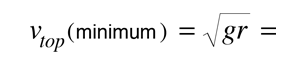# Motion in a Vertical CircleThe motion of a mass on a string in a vertical circle includes a number of mechanical concepts. It must satisfy the constraints of centripetal force to remain in a circle, and must satisfy the demands of conservation of energy as gravitational potential energy is converted to kinetic energy when the mass moves downward. The velocity must increase as the mass moves downward from the top of the circle, subject to the constraints stated.

For a mass moving in a vertical circle of radius r = m,

if we presume that the string stays taut, then the minimum speed for the mass at the top of the circle is (for g = 9.8 m/s2)m/s

This is the condition for "weightlessness" in any curved motion in a vertical plane. For any velocity above this minimum, we can use conservation of energy to relate the velocity at the bottom of the circle to the velocity at the top.

For a velocity at the top vtop = m/s

the velocity at the bottom is vbottom = m/s

Using the centrifugal force conditions, the tension at the bottom can be related to the tension at the top:For a mass m = kg,

the tension at the top of the circle is Ttop = Newtons.

The corresponding tension at the bottom of the circle is Tbottom = Newtons.

Index

Rotational motion

 HyperPhysics***** Mechanics R Nave
Go Back